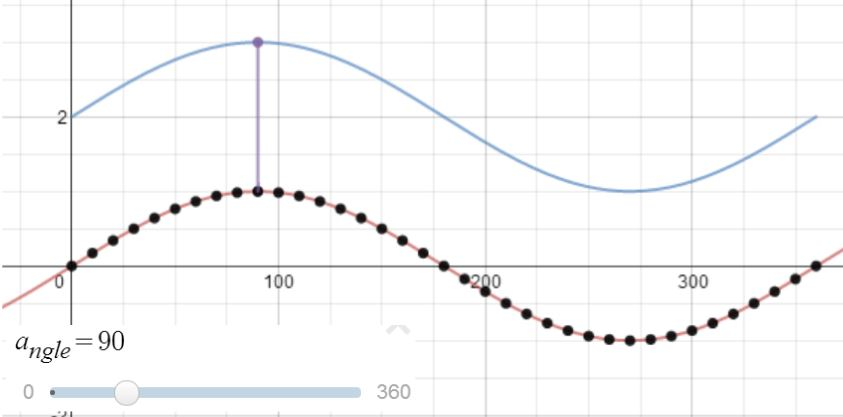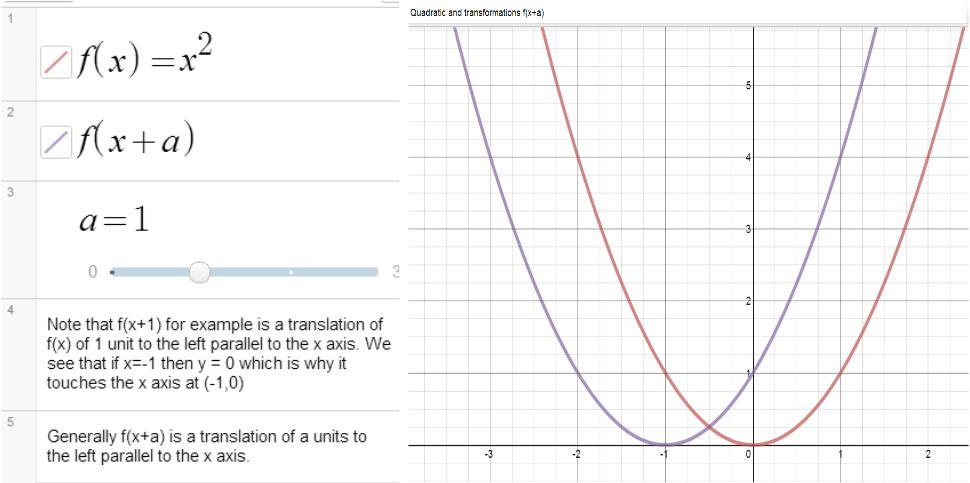# It started with a tweet!I noticed a tweet from Darren Kuropatwa..Here is what Darren was referring to, a video made by Desmos on exploring the sine function:

Darren has recreated that graph here.

Now I rather like that and thinking it would be useful for my revision session this week with a GCSE (UK age15-16) class, decided I would simplify it so it was more suitable for my students. I changed  the units to degrees and restricted the transformations more so it was more in line with our specification (these students are studying for a second GCSE in Mathematics – AQA Further Mathematics level 2).

Along came Desmos having seen the Twitter conversation..and look at the awesome graph Desmos created which shows a sine curve and a transformed curve clearly illustrating how each point is changed.

We could for example translate the curve 2 units parallel to the y axis:Using the slider for angle we see very clearly that each y coordinate is increased by 2.

Brilliant!

Thank you Desmos (and Darren!)

# Graphs and TransformationsNote how easy it is using the Desmos graphing calculator to show a graph and then the same graph after a transformation. For example see here the graph of x2 and (x+a)(or click on  the image).

As you can see all you need do is define f(x) which then gives you the ability to graph transformations of f(x). Using a slider means students can explore what happens if the value of a changes. In this particular example a is restricted to integer values from 0 to 3 but this is very easy to change by editing the slider; simply click on the numbers at the ends of the slider and choose the values required, you can also choose the step size.

I like the ability to add text to graph pages which means it is possible to add a few words of explanation or even questions for students.

I have used these prompts before in a computer room, the aim being for students to explore and generalize. The headings are links to Desmos graph pages students could experiment with.

Transformations Exercise

For linear, quadratic and the sine and cosine functions the following transformations should be understood:

y=f(x)+a trigonometric
Eg plot y = x2   and y = x+ 3   on the same diagram, compare the graphs.
Plot y = x− 4, y = x− 1
Compare y = x2  and y = x+ k where k is any integer, positive or negative.
Also compare y = sin x and y = sin x + 3.
Compare y = sin x and y = sin x + k where k is any number

y = f(x+a) trigonometric
Eg plot  y = x2   and y = (x+ 3)on the same diagram, compare the graphs.
Compare y = x2   and y = (x−3)2.  Compare y = x2   and y = (x−4)2
Compare y = x2  and y =(x+ k)2 where k is any integer, positive or negative.
Compare y = sin x and y = sin (x+90) , sin (x – 90), sin(x+180)
Compare y = cos x with y = cos(x+90), cos (x-90)

3. y = af(x) trigonometric
Compare y = sinx and y = 2sinx.
Compare y = cosx and y = 3cosx and y =  0.5cos x
Compare y=x2 and kx2 where k is any integer positive or negative.

4. y = f(ax) trigonometric
Compare y = sinx and y = sin2x. Compare y = sin x and y = sin(0.5x).
Compare y = cos x and y = cos 3x. Compare y = cos x and y = cos(0.5x)